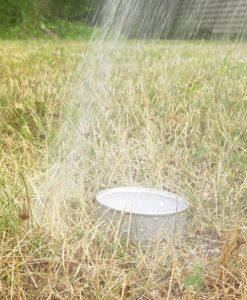Landscape Report

# What is an Inch of Water?The unpredictable Midwest weather has gardeners checking their rain gauge daily, because one rule gardeners learn early on is that landscapes need about an inch of water each week. Of course, there are many factors that will impact the accuracy of this very general rule such as soil type, average temperature, sun exposure, plant type, and wind. The crud method to determine whether you need to water would be poking your finger in the soil and if it feels dry, you water. But what is an inch of water really?

To determine this, we have to go back to the basics of geometry to measure area and volume. However, a few measurements will always remain the same.

One square foot = 144 square inches

One gallon = 231 cubic inches

Therefore, an “inch of water” is 0.62 gallons per square foot of garden area. Unless you use a gallon jug to water a square foot garden, this number may still leave you wondering how to obtain that inch of water or 0.62 gallons per square foot. Of course, this will vary depending on the type of irrigation used, but the total amount of water needed for an area will remain constant. First, determine the garden area in square feet and then multiply the total area by 0.62 gallons.

Example 1:        The garden is 10 feet wide and 20 feet long, so 20 ft × 10 ft = 200 square feet.

Then, 200 ft2 × 0.62 gallons = 124 gallons needed for a 200 ft2 garden area.

There are a few ways to measure irrigation water and some will include acronyms like ac.in, psi, or GPM. Before trying to convert an acre-inch (ac.in) or determining pounds per square inch (psi), grab a one-gallon bucket and a stop watch. Time how long it takes to fill the bucket using your preferred spray nozzle. Then, divide 60 by the number of seconds that it took to fill the one-gallon bucket. This equals the gallons per minute (GPM).

Example 2:        The one-gallon bucket fills in 20 seconds, so 60 ÷ 20 = 3 gallons per minute.

Next, to determine how long to water using this spray nozzle, divide the total amount of water needed for the whole garden area (from Example 1) by the gallons per minute calculated (from Example 2).

Example 3:        124 gallons needed ÷ 3 gallons per minute = 41.3 minutes of watering.

In the above scenario, the garden area would need to be watered for approximately 40 to 45 minutes each week. Ideally, this would be broken into two watering sessions per week, so about 20 minutes every few days. If watering with a spray nozzle for that length of time isn’t possible, gardeners may decide to switch to a sprinkler or a soaker hose. This will require a new calculation and a few tuna cans.Figure 1

When irrigation with a sprinkler is necessary, it is important to measure the water output to avoid over or under-watering areas. This is another simple measurement using a few tuna cans, a ruler, and a stop watch. Tuna cans are convenient, but any short container with a wide mouth and straight sides can be used. Place the empty containers randomly throughout the area that is being irrigated and turn the sprinkler on for 10 minutes (Fig. 1). Using the ruler, measure how much water is in each container and calculate the average.

Example:          Three cans were placed in the garden and collected the following amounts of water in 10 minutes: 0.5”, 0.75”, and 0.75”. The average is the sum divided by the number of cans. So, (0.5+0.75+0.75) ÷ 3 = 0.67 inches of water every 10 minutes.

Once these calculations are complete, you can confidently water your garden with approximately an inch of water each week. Keep in mind that numerous other factors can impact whether you need more or less water, so watch the thermometer and wind speeds. High temperatures and high winds can dry out soils very quickly, even a well-watered garden.